#2018 iT 邦幫忙鐵人賽DAY 17
2
Modern Web

## [PixiJS - Day-17] tint 上色與在 PixiJS 玩顏色

(設定 `tint:0xFFFFFF` 會移除套色效果)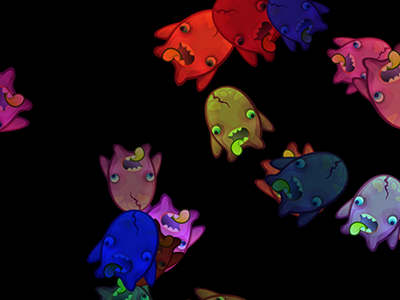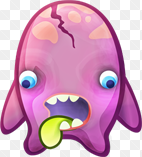tint 可以把指定的顏色套到物件上

PIXI.Container：`不支援`
PIXI.Sprite：`支援`
PIXI.extras.AnimatedSprite：`支援`
PIXI.Graphics：`支援`
PIXI.Text：`支援`

Wiki: HSL和HSV色彩空間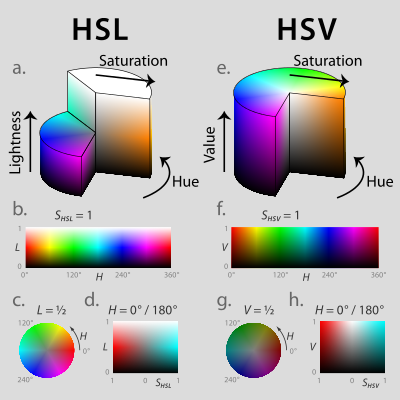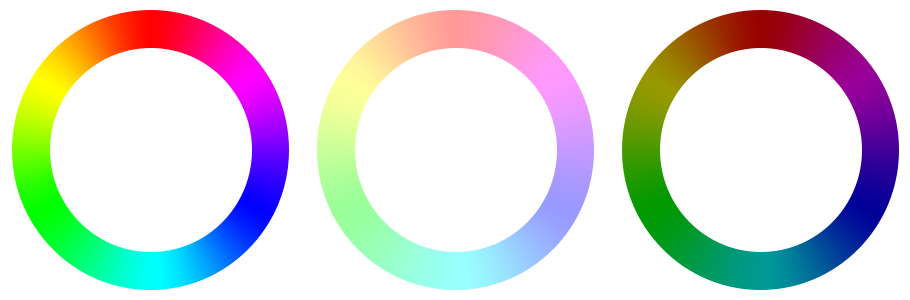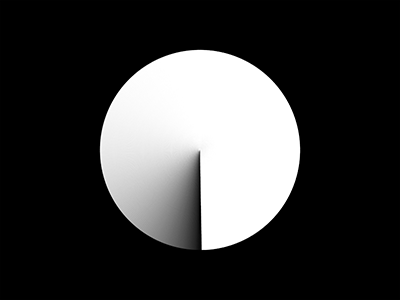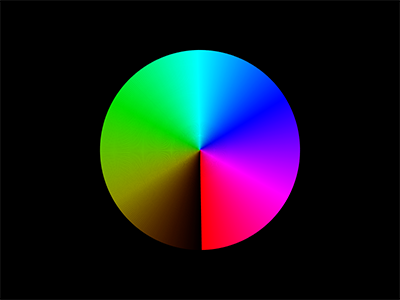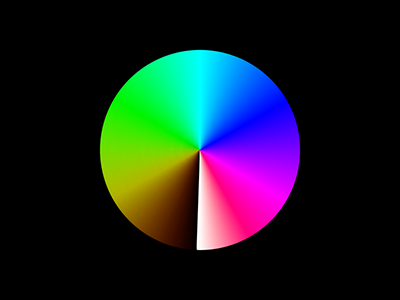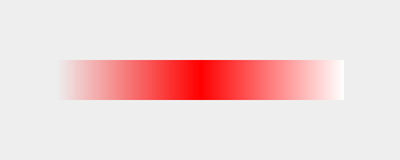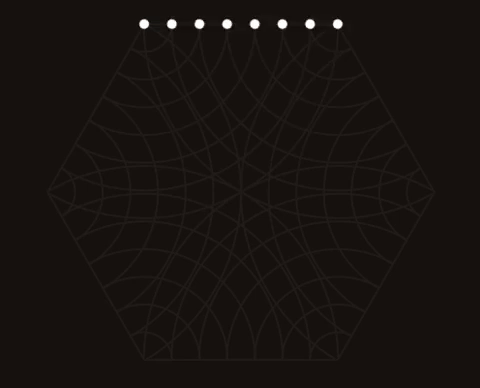(原圖為網路上一張 Gif，我使用 PixiJS 練習重製出一樣的效果)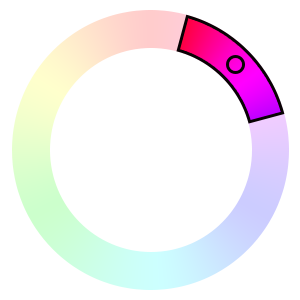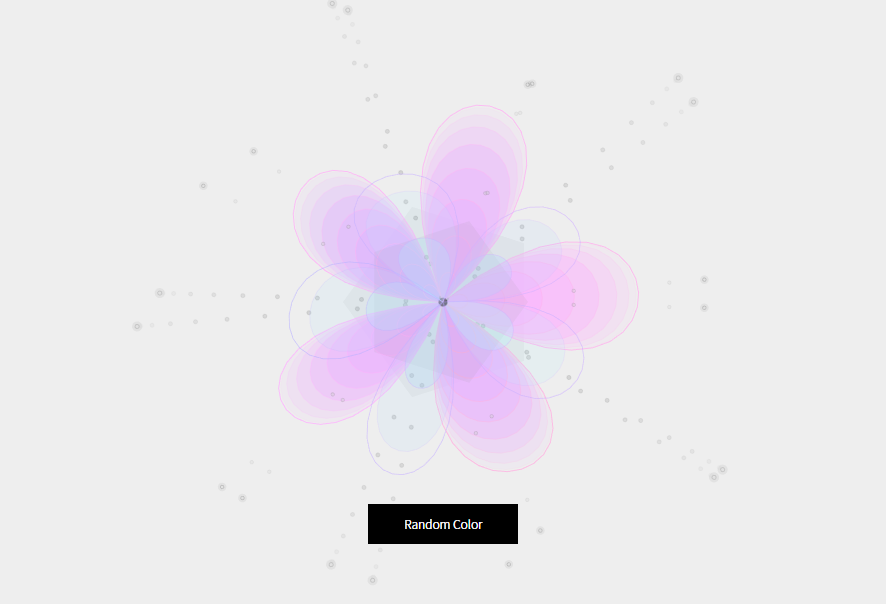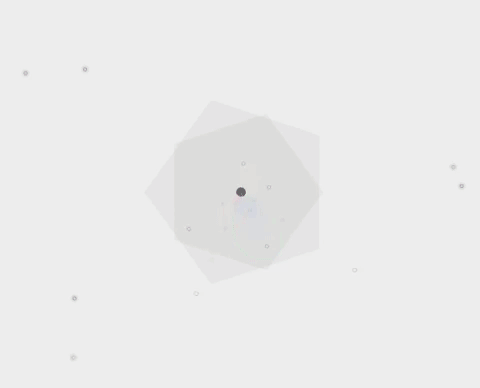### 1 則留言

0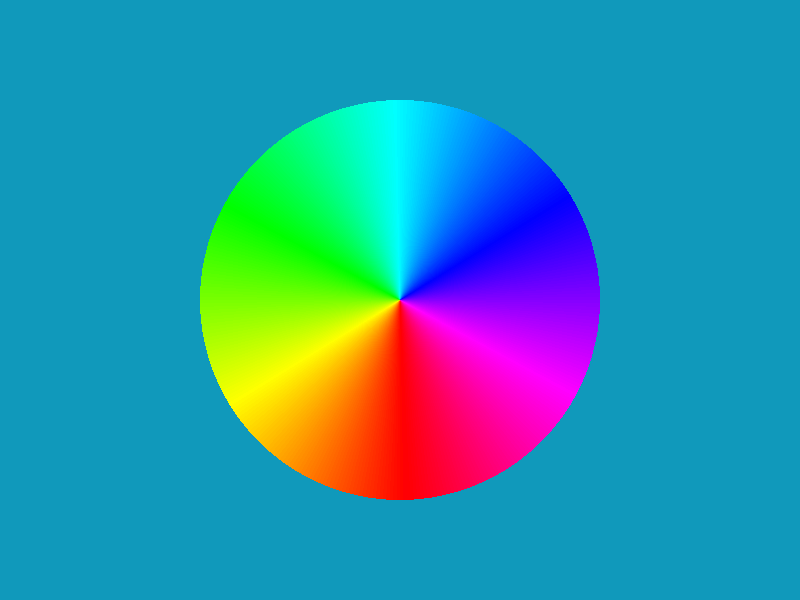``````var t = new PIXI.Graphics();
var totalCount = 360;
for(var i = 0;i< totalCount; i++){
var colorArray = hsvToRGB2( i * 360 / totalCount, 1, 1);
var color = colorArray * 65536 + colorArray * 256 + colorArray;

var arcGraphic = new PIXI.Graphics();

// 畫扇形的扇部
arcGraphic.beginFill(color, 1);
arcGraphic.arc( 0, 0, 200, (0) * (Math.PI / 180), (1) * (Math.PI / 180) );
arcGraphic.endFill();

// 畫扇形的三角形部分
arcGraphic.beginFill(color, 1);
arcGraphic.moveTo(0, 0);
arcGraphic.lineTo(0, 200);
arcGraphic.lineTo(Math.sin( 2 * (Math.PI / 180)) * 200, Math.cos( 2 * (Math.PI / 180)) * 200);
arcGraphic.lineTo(0, 0);
arcGraphic.endFill();

arcGraphic.rotation = i * (Math.PI / 180);
}
``````

tween 的部分滿硬的，內容偏向用程式製作動畫

zxcv13579 iT邦新手 5 級 ‧ 2018-01-08 10:58:07 檢舉